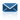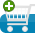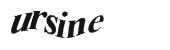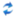+91-9818369374Ask an Expert for Free Submit Your Assignment/Home work1 Live Tutoring Free Trial / 30 Min Sample Assignment / Home Work Make Payment Course Offered Resources
Quick Contact# Question

Cardano (Italian mathematician in the 16th century) came up with the following problem:

The sum of two numbers is 10 and the product is 40, what are the two numbers?

# IB Math HL – Complex Numbers

Cardano (Italian mathematician in the 16th century) came up with the following problem:

The sum of two numbers is 10 and the product is 40, what are the two numbers?

Rafael Bombelli wrote a book on Algebra 15 years after Cardano, including the following example:Cardano published a formula that another Italian mathematician, Del Ferro, had come up with in 1545, for the solution of an equation of the form, the solution being:Using this formula, the solution to Bombelli’s example is:Bombelli decided that the value of this should be 4 (the only real solution), and that the two cube root expressions should be equivalent toand, the sum of which would be 4.

This striking thought process can be seen as the birth of what we know today as complex numbers.

Not only mathematics, but also science and technology have benefitted from these new abstract elements.is given the letter i (imaginary unit)andComplex numbers have the form, where. Calculating with complex numbers works with the same principles as real numbers.

Examples:

a.b.Special case (why?):This is handy when dividing by complex numbers.

Examples:Powers of complex numbers can also be calculated, for example:With________, we look back at Bombelli who decided thatwas equivalent to…….

So, now we have a new addition to our world of numbers………………

Are complex numbers a subset of real numbers, or are real numbers a subset of complex numbers, or neither?

Complex numbers are consistent with the rules of numbers as we know them for real numbers, except for the rules for inequalities. Why is this so?

SOURCE:

‘Wiskunde in een Notendop’ by Martin Kindt and Ed de Moor

(Publ. Uitgeverij Bert Bakker) 2008

AssignmentWork Payment (USD)
Content